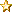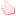###Author Topic: AnalogInput units  (Read 338 times)

#### Nick_W

• Full Member
•• Posts: 214« on: February 06, 2017, 09:18:21 am »
I'm reading an analogInput pin, and I would like to convert the reading from mV to voltage independent units (ie 0-1023), as the battery voltage may vary.

I'm currently assuming that reading the Battery Voltage will give me the maximum value (1023 units) and using the following to convert:

Code: [Select]
`((1023 * reading) / batvolt).ToInteger()`
However it occurs to me that this cannot in fact be correct (or the battery voltage would always read maximum), so there must be an internal voltage reference.

The documentation does say this "When a pin is in AnalogInput mode, the system reads the value based on the internal band-gap reference value."

Reading the data sheet, the internal reference is 0.6V, so assuming a gain of 1/6 this would give a range of 0-3.6V (which seems to be the case).

This would then make my conversion:
Code: [Select]
`((1023 * reading) / 3600).ToInteger()`
Is this correct? am I making the correct assumptions here?

Thanks.

#### mc-John

• Global Moderator
• Full Member
•• Posts: 212##### Re: AnalogInput units
« Reply #1 on: February 06, 2017, 06:59:41 pm »
We have the resolution at 14-bit. The system returns the result (NRF_SAADC->RESULT.PRT). Because we return the value in millivolt we multiply that by 3600 and divide that by 2^14.

Code: [Select]
`return (result*3600)>>14;`

To get the value back you just have to undo this like the code below:

Code: [Select]
`result = (millivolt<<14)/3600;`

#### Nick_W

• Full Member
•• Posts: 214##### Re: AnalogInput units
« Reply #2 on: February 07, 2017, 07:01:33 am »
OK, so your reference is 3600 though, not BatVolt as I suspected.

I'll stick with what I have, no-one needs 14 bits from an on-board ADC, I need 10, same as most applications out there.

BTW, don't you have to oversample to get 14 bits? the built in is only 12 bits. That would slow down the response quite a bit, and seems somewhat pointless. Why not stick with 12 bits? it's more than most applications could possibly need, no-one expects nanoVolt precision from these devices.

#### mariahernandez

• Newbie
•• Posts: 2##### Re: AnalogInput units
« Reply #3 on: March 21, 2017, 03:47:29 pm »
Hello mcThings' team,

I'm working on a energy sensor using the mc-module120, at the first time when I did't know the resolution of the mc-Module I decided to power the analog input to get the resolution of it and I found that if I power the module with 2,8V(using the VCC pin of the mc-Module) I got a value range close to 2600, and if I power it with 3.3V (external power supply) I got a lower result value close to 1700. That's no make too much sense for me, because if I power the module with a highger voltage the result should be highger and not less, or not? e.i. If I power an Analog input of a NodeMCU with 3.3V I get that the resolution is 1024 (10 bits resolution)

After reading this post I found the that the resolution of the mc-module is 16384 (14 bits resolution), but if I use the following formula (using the correct resolution) I'm not able to get the correct value from the sensor.

Code: [Select]
`value = (sensorReading*3.3)/16384;`
At the moment I have the energy sensor working with a Particle Electron working perfectly. The resolution of this device is 12 bits (4096) and with the following formula is how I get the value from the sensor:

Code: [Select]
`float value = (analogRead(A0)*3.3)/4096.0;`
Btw, I'm using a i-Snail-VC-50 -> http://www.phidgets.com/products.php?product_id=3502 if you know something about it I would appreciate any help!

I hope you can solve my doubts with the resolution of the module as soon is possible to start working with it, I'm so excited to see it working!

Best Regards,
Maria C

#### Nick_W

• Full Member
•• Posts: 214##### Re: AnalogInput units
« Reply #4 on: March 21, 2017, 04:28:02 pm »
Maria,

I think you are mixing float and integer types, plus the voltage reference is 3600 not 3.3.

What I think you are looking for is:

Code: [Select]
`value = (sensorReading\3600)*16384`
or for 0-1024
Code: [Select]
`value = (1023 * reading) \ 3600`
where "value" is an integer type. Due to some odd math in McThings, division (/) always returns a float, so unless "value" was a float, you would get strange results. To do integer division, you use \ as shown above.

This will give you a reading from 0 to 16384 (0 to 3.6V), or 0-1024 if you use the second version. 3.6V is the maximum you can read. Note: this is not 3.3V or battery voltage, but is based on the internal 0.6V reference - so you can't change it, or use another number!

You also need to keep your units and data types consistent, sensorReading is in mV, and is a Short, 3.3 is in Volts and is a float. This combination is probably causing all the headaches.

Good luck with the project!
« Last Edit: March 21, 2017, 04:37:06 pm by Nick_W »

#### mariahernandez

• Newbie
•• Posts: 2##### Re: AnalogInput units
« Reply #5 on: March 21, 2017, 06:04:25 pm »
Hey Nick,

Thanks for clearing out the variables types and the division returns, I'm going to modify the code and test it again!

But I'm still a little confused about the readings. I've cleared that the reading is 0 to 16384 (0 to 3.6V) but if I apply 3.3V to the analog pin I should get a value close to the 16384 or not?  This is the point that I get confused; when I run one test at the lower voltage I get a highger resulting value, example: 2.8V = 2600 | 3.3V =1700 (doesn't make sense for me).

Which second version are you talking about?

Thanks so much for the support!

#### Nick_W

• Full Member
•• Posts: 214##### Re: AnalogInput units
« Reply #6 on: March 22, 2017, 07:50:33 am »
Maria,

OK I'm confused by what you mean by "reading". If you just take the value from the analog pin (no math whatsoever), you get the voltage in mV. ie 2.8V = 2800, 3.3V = 3300 etc. up to 3600 (3.6V) which is the maximum you can read. You can read this as a short or an integer.

I am doing this in several situations, and it works exactly as predicted. I haven't tried reading higher than the supply voltage though.

The problem is that having the value in mV is not very useful, as you have to translate it into some sort of meaningful number, and most libraries/code expects units, not mV.

The two formulae's I gave you convert the mV to units. One does 0-16384 (14 bit) the other 0-1024 (10 bit). it's simple, divide the analog pin value (mV) by the reference value (3600) and multiply by whatever you want as full range, to get the arduino-style number. Just keep your units and data types straight. ie 3600 (not 3.3), integer division (not float), in integer maths you multiply before you divide, to avoid rounding errors, and so on.

Just FYI, on your particle electron, analogRead() reads units, not volts. On the mc120, analogRead() returns volts, not units. It's the other way round. I think this is the root of most of your confusion.

If you can post your whole code, maybe I can spot what is going wrong...
« Last Edit: March 22, 2017, 08:05:31 am by Nick_W »Like x 1 View List